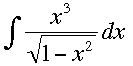SEARCH HOMEMath Central Quandaries & QueriesQuestion from William, a parent: The integral of X^3/the square root of 1-x^2 dx. Can you please find the absolute value of x if x is less than 1. I do need to have an answer by monday (3/8) at 9am. Thanks in advance for your help.William,

I can get you started on the integralLet x = sinθ then dx = cosθ dθ.

Substitute into the integral and simplify. Can you integrate the resulting expression?

Write back if you need more help,
HarleyMath Central is supported by the University of Regina and The Pacific Institute for the Mathematical Sciences.